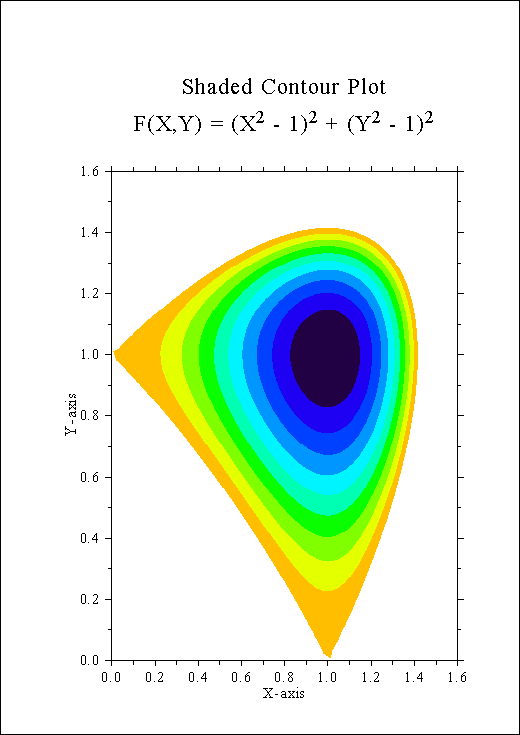## B.5 Shaded Contour Plot

```%GCL
// Shaded Contour Plot

N = 100
FLOAT ZMAT[N,N], XRAY[N], YRAY[N], ZLEV

STEP = 1.6 / (N - 1)
XRAY = FALLOC (N) * STEP
YRAY = FALLOC (N) * STEP
X    = XRAY * XRAY - 1

DO J = 0, N - 1
Y=YRAY[J] * YRAY[J] - 1.
ZMAT[*,J] = X * X + Y * Y
END DO

METAFL ('CONS')
SETPAG ('DA4P')
DISINI ()
COMPLX ()
PAGERA ()

NAME   ('X-axis', 'X')
NAME   ('Y-axis', 'Y')

MIXALF ()
TITLIN ('Shaded Contour Plot', 1)
TITLIN ('F(X,Y) = (X[2\$ - 1)[2\$ + (y[2\$ - 1)[2\$', 3)

SHDMOD ('POLY', 'CONTUR')
AXSPOS (450, 2670)
GRAF   (0., 1.6, 0., 0.2, 0., 1.6, 0., 0.2)

HEIGHT (30)
DO I = 0, 11
ZLEV[11-I] = 0.1 + I * 0.1
END DO

CONSHD (XRAY, N, YRAY, N, ZMAT, ZLEV, 12)

HEIGHT (50)
TITLE  ()
DISFIN ()
```Figure B.5: Shaded Contour Plot

Next | Previous | Contents